biomcmc-lib  0.1 low level library for phylogenetic analysis
prob_distribution.h File Reference

Probability distribution functions and auxiliary mathematical functions from statistical package R. More...

#include "lowlevel.h"
#include "random_number.h"
Include dependency graph for prob_distribution.h: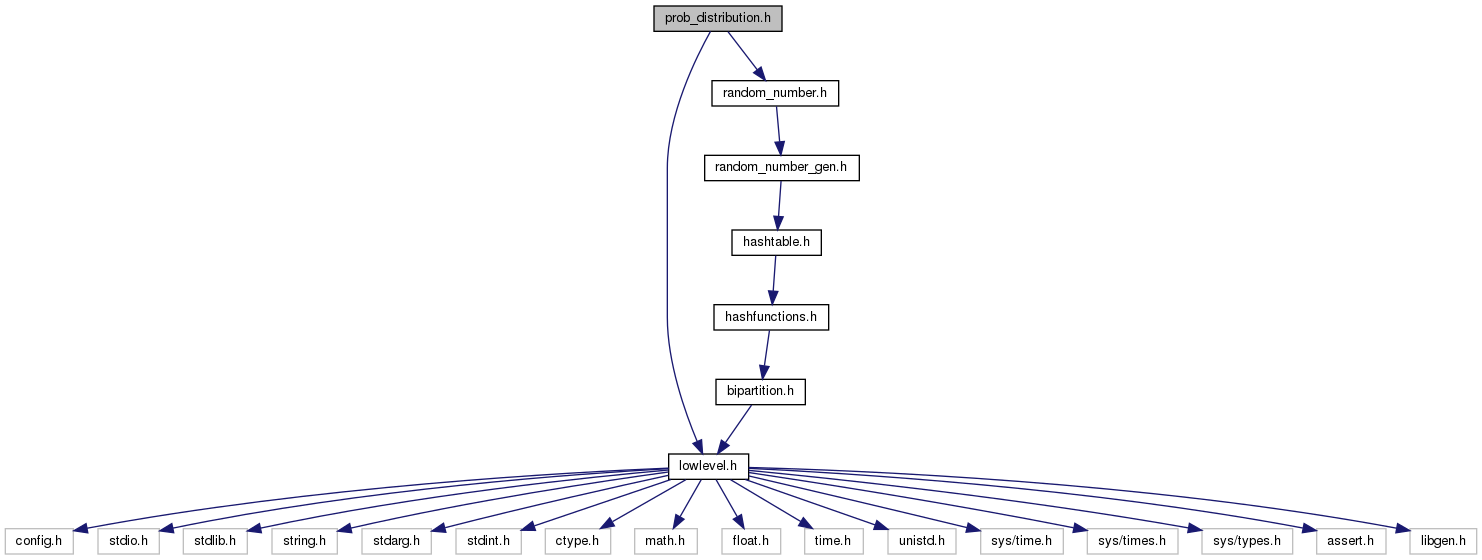This graph shows which files directly or indirectly include this file: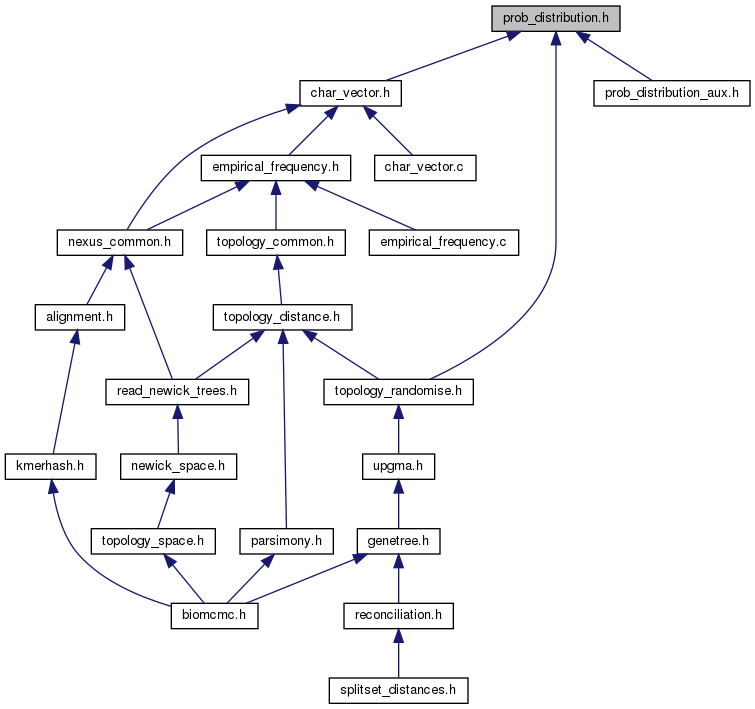Go to the source code of this file.

## Data Structures

struct  discrete_sample_struct

## Typedefs

typedef struct discrete_sample_structdiscrete_sample

## Functions

void biomcmc_discrete_gamma (double alpha, double beta, double *rate, int nrates)
Ziheng Yang's gamma discretization of rates.

double biomcmc_dexp_dt (double d, double lambda, double m, bool log_p)
pdf of discrete truncated exponential (d is discrete, m is maximum value) More...

double biomcmc_pexp_dt (double d, double lambda, double m, bool log_p)
cdf of discrete truncated exponential (d is discrete, m is maximum value): calculates P(D <= d)

double biomcmc_qexp_dt (double p, double lambda, double m, bool log_p)
quantile of discrete truncated exponential, that is, finds d s.t. P(D <= d) >= p

double biomcmc_dgamma (double x, double alpha, double beta, bool log_p)
gamma density

double biomcmc_qgamma (double p, double alpha, double beta, bool log_p)
gamma quantile (inverse CDF)

double biomcmc_pgamma (double x, double alpha, double beta, bool log_p)
computes the cummulative distribution function for the gamma distribution with shape parameter alpha and rate parameter beta, s.t.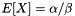. The same as the (lower) incomplete gamma function.

double biomcmc_dnorm (double x, double mu, double sigma, bool log_p)

double biomcmc_qnorm (double p, double mu, double sigma, bool log_p)

double biomcmc_pnorm (double x, double mu, double sigma, bool log_p)

double biomcmc_dlnorm (double x, double meanlog, double sdlog, bool log_p)

double biomcmc_qlnorm (double p, double meanlog, double sdlog, bool log_p)

double biomcmc_plnorm (double x, double meanlog, double sdlog, bool log_p)

double biomcmc_dpois (double x, double lambda, bool log_p)

double biomcmc_qpois (double p, double lambda, bool log_p)

double biomcmc_ppois (double x, double lambda, bool log_p)

double biomcmc_rng_gamma (double alpha, double beta)

double biomcmc_rng_norm (double mu, double sigma)
Returns a random number from a Normal distribution N(mu, sigma^2) using 52 bits of precision.

double biomcmc_rng_lnorm (double meanlog, double sdlog)

double biomcmc_rng_pois (double mu)

double biomcmc_lgammafn (double x, int *sgn)

double biomcmc_gammafn (double x)

double biomcmc_log1p (double x)
compute the relative error logarithm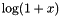(C99 standard)

double biomcmc_log1pmx (double x)
accurate calculation of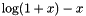, particularly for small x

double biomcmc_expm1 (double x)
compute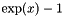accurately also when x is close to zero, i.e.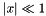discrete_sample new_discrete_sample_from_frequencies (double *prob, size_t size)

void del_discrete_sample (discrete_sample g)

size_t biomcmc_rng_discrete (discrete_sample g)

double biomcmc_discrete_sample_pdf (discrete_sample g, size_t k)

double biomcmc_logspace_add (double logx, double logy)

double biomcmc_logspace_sub (double logx, double logy)

bool biomcmc_isfinite (double x)
check if number is between minus infinity and plus infinity, or NaN

## Detailed Description

Probability distribution functions and auxiliary mathematical functions from statistical package R.

Code derived from the R project for Statistical Computing version 2.9.1, available under the GPL license. It might be possible to use directly the standalone mathematical library "Rmath.h" from the R project instead of our implementation. The advantage would be a library updated more often than mine, at the cost of delegating to the guenomu user the installation and maintenance of the extra libraries (like GSL, for instance). In Debian this library can be installed through the package "r-mathlib". The original R library checks for several built-in compiler functions (like log1p(e) for calculating log(e+1) ) but I simply assume the compiler has none and reimplement them. The CDFs always assume the lower tail (upper tails must use 1. - lower tail) or equivalent.

The code for the discrete sampling comes from the GNU Scientific Library version 1.14

## ◆ biomcmc_dexp_dt()

 double biomcmc_dexp_dt ( double d, double lambda, double m, bool log_p )

pdf of discrete truncated exponential (d is discrete, m is maximum value)

pdf of discrete truncated exponential (d is discrete, m is maximum value)